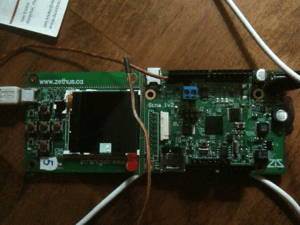# Basic Arduino Support for Gina BoardI’ve built in basic support for the Arduino 1.0RC1 for the Gina Boards. This is the modified version of the “Blink sketch” running.  No big deal here, but this will make the board more accessible to those not interested in programming details.

`int ledPin1 =  13; `
`int ledPin2 =  14; `
`int ledPin3 =  15; `
`int ledPin4 =  16; `
`// The setup() method runs once, when the sketch starts`
`void setup()   {`
`// initialize the digital pin as an output:`
`pinMode(ledPin1, OUTPUT);`
`pinMode(ledPin2, OUTPUT);`
`pinMode(ledPin3, OUTPUT);`
`pinMode(ledPin3, OUTPUT);`
`}`
`// the loop() method runs over and over again,`
`// as long as the Arduino has power`
`void loop()`
`{`
`digitalWrite(ledPin1, HIGH);   // set the LED on`
`delay(200);                  // wait for a second`
`digitalWrite(ledPin2, HIGH);    // set the LED off`
`delay(200);`
`digitalWrite(ledPin3, HIGH);    // set the LED off`
`delay(200);`
`digitalWrite(ledPin4, HIGH);    // set the LED off`
`delay(200);`
`digitalWrite(ledPin1, LOW);   // set the LED on`
`delay(100);                  // wait for a second`
`digitalWrite(ledPin2, LOW);    // set the LED off`
`delay(100);`
`digitalWrite(ledPin3, LOW);    // set the LED off`
`delay(100);        // wait for a second`
`digitalWrite(ledPin4, LOW);    // set the LED off`
`delay(100);        // wait for a second`
`}`